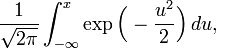# Why Do We Use Numerical Methods?

Numerical methods are techniques to approximate mathematical procedures (an example of a mathematical procedure is an integral).

Approximations are needed because we either cannot solve the procedure analytically (an example is the standard normal cumulative distribution function)or because the analytical method is intractable ( an example is solving a set of a thousand simultaneous linear equations for a thousand unknowns for finding forces in a truss)_______________

This post is brought to you by

• Holistic Numerical Methods Open Course Ware:
• the textbooks on
• the Massive Open Online Course (MOOCs) available at